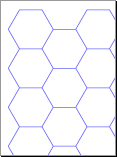## Hexagonal Graph Paper PDF Generator

Line Thickness:
points
Hexagon Size:
Put a dot in the middle of each hexagon
Color:
Hex #8.5" x 11"
x
Margin:

### Calculating various bits about regular hexagons

Given length of a side x...
Tip to tip across the hex is 2x.
Height of the hex flat side to flat side is 2x(sqrt(3/4)) or about 1.732x.
Area of the hex is 1.5(x^2 (sqrt(3)) or about 2.56x^2.

Example: Making graph paper with 4 hexes per square inch
Hexagon with a side length of x... The area of that hex would be about...
2.6 (x^2)
So for 4 hexes per square inch...
4 * 2.6 (x^2) = 1
x^2 = 1/10.4
x^2 = .096
x = .31 inches per side.

Extra: 1 sq. in. per hex ~= 0.6204

## Customized Output Formats

If you're looking for a program to generate labeled hex grids in other formats (such as PNG or SVG images), check out mkhexgrid.
It has some additional options for labeling your grid as well. Last updated over a decade ago, but how much do hexagons change?